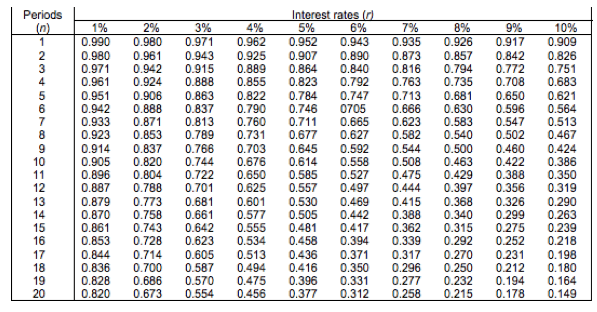Present Value Table

What is a Present Value Table?

Definition: A present value (PV) table allows you to convert a future sum, or a stream of money to be received at regular intervals in the future, into its current value. It does this by providing various coefficients – a number with which you have to multiply the future cash flow(s) – to arrive at the present value.

What does Present Value Table mean?

A PV table is made up of multiple rows and columns. The column on the left indicates periods in weeks, months, or years. The row at the top indicates the different discount rates. When you are using a PV table you need to select the coefficient that matches your requirement. To understand this, let us view a PV table:By using this table, you can calculate the present value of a sum of money that you will receive at a future date. Of course, any such calculation will have to take a certain rate of interest into account.

If you require \$50,000 in exactly five years from now, how much money should you put into a bank account that pays an interest rate of 3% on an annual basis?

You can answer this question by looking up the coefficient under the 3% column for row #5. The coefficient of 0.863 multiplied by \$50,000 yields \$43,150, which is the sum that you need to invest.

Remember that the result that you obtain by using a PV table will not be as accurate as the answer that you will get with a financial calculator. That’s because the coefficient is rounded off to three digits in the PV table that we have used.

Example of Present Value Table

The owner of Bryan’s Retreat, a popular restaurant, receives an offer from a potential buyer who is willing to pay \$1 million after one year. The current market value of Bryan’s Retreat is \$700,000.

If the owner of Bryan’s retreat uses a discount rate of 9% to calculate the present value of the \$1 million offer, he arrives at a figure of \$917,000 (\$1 million X 0.917). That’s significantly more than the restaurant’s current market value. If the other terms of the offer meet the owner’s requirements, he should go ahead with the sale.

Summary

A PV table is a substitute for a financial calculator or for computer software. It can be used to calculate a figure’s present value by simply multiplying it by the appropriate coefficient.

Get the latest CPA news and discounts!

=Get My 4 Resources I Used to Pass the CPA Exam on My 1st Try!

*We promise never to sell your information or send you spam